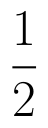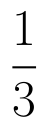Select Page

# 12 Science CBSE Answers for MCQ Maths Relations and Functions in English

12 Science CBSE Answers for MCQ Maths Relations and Functions in English to enable students to get Answers in a narrative video format for the specific question.

Expert Teacher provides 12 Science CBSE Answers for MCQ Maths Relations and Functions through Video Answers in English language. This video solution will be useful for students to understand how to write an answer in exam in order to score more marks. This teacher uses a narrative style for a question from Relations and Functions not only to explain the proper method of answering question, but deriving right answer too.

Please find the question below and view the Answer in a narrative video format.

Question:

## Similar Questions from CBSE, 12th Science, Maths, Relations and Functions

Question 1 :  Letdefined as f(x) = 5 be a constant function. Then its range is (View Answer Video)

Question 2 : Ifis defined by, write f(f(x)). (View Answer Video)

Question 3 : Let A = {1, 2, 3}. Then, number of equivalence relations containing (1, 2) are, (View Answer Video)

Question 4 : The number of binary operations which can be defined on the setis, (View Answer Video)

Question 5 : Let * be the binary operation on N given by a * b = LCM of a and b. Find 5 * 7. (View Answer Video)

### Application of Integrals

Question 1 : Using the method of integration find the area of the region bounded by lines:
2x + y = 4, 3x - 2y = 6 and x - 3y + 5 = 0.

Question 2 : Using integration find the area of region bounded by the triangle whose vertices are (-1, 0), (1, 3) and (3, 2). (View Answer Video)

Question 3 : Find the area of the region enclosed by the parabolathe line y = x + 2. (View Answer Video)

Question 4 : Using integration, find the area of theco-ordinates whose vertices are P(2,0), Q(4, 5) and R(6,3). (View Answer Video)

Question 5 : Using integration, find the area of the region bounded by the triangle whose vertices are (-1, 2), (1, 5) and (3, 4). (View Answer Video)

### Probability

Question 1 : A box contains 50 bolts and 50 nuts. Half of the bolts and nuts are rusted. If two items are drawn with replacement, what is the probability that either both are rusted or both are bolts.    (View Answer Video)

Question 2 :  Probability of solving specific problem independently by A and B areandrespectively. If both try to solve the problem, independently, then find the probability that exactly one of them solves the problem.  (View Answer Video)

Question 3 : Three cards are drawn without replacement from a pack of 52 cards. Find the probability that the cards drawn are king, queen and jack.